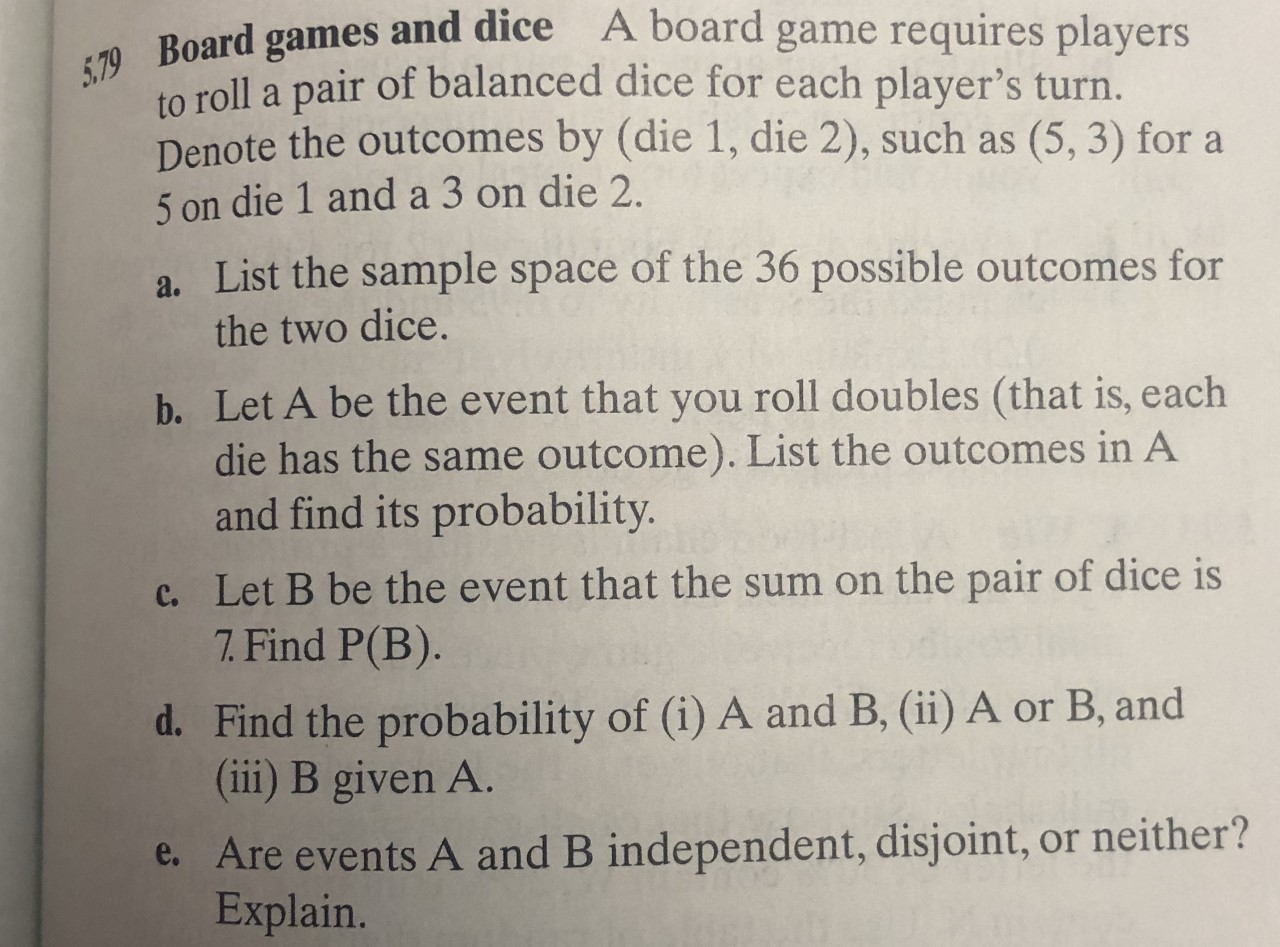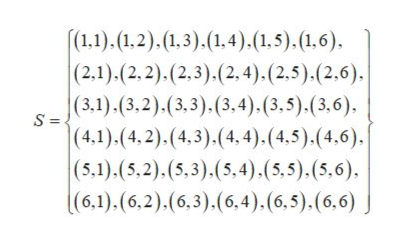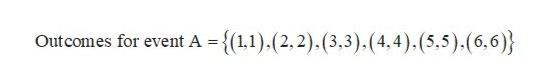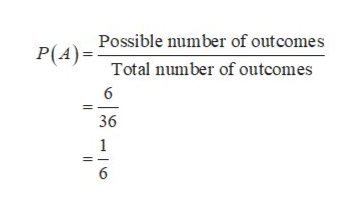A board game requires players79 Board games and diceto roll a pair of balanced dice for each player's turn.Denote the outcomes by (die 1, die 2), such as5 on die 1 and a 3 on die 2.(5, 3) for aa. List the sample space of the 36 possible outcomes forthe two dice.b. Let A be the event that you roll doubles (that is, eachdie has the same outcome). List the outcomes in Aand find its probability.c. Let B be the event that the sum on the pair of dice is7. Find P(B).d. Find the probability of (i) A and B, (ii) A or B, and(ii) B given A.e. Are events A and B independent, disjoint, or neither?Explain.

Question

Part D and Ehelp_outlineImage TranscriptioncloseA board game requires players 79 Board games and dice to roll a pair of balanced dice for each player's turn. Denote the outcomes by (die 1, die 2), such as 5 on die 1 and a 3 on die 2. (5, 3) for a a. List the sample space of the 36 possible outcomes for the two dice. b. Let A be the event that you roll doubles (that is, each die has the same outcome). List the outcomes in A and find its probability. c. Let B be the event that the sum on the pair of dice is 7. Find P(B). d. Find the probability of (i) A and B, (ii) A or B, and (ii) B given A. e. Are events A and B independent, disjoint, or neither? Explain. fullscreen
Step 1

a. The sample space of the 36 possible outcomes for the two dice is,help_outlineImage Transcriptionclose(1,1).(1,2).1,3).(1.4). (1,5). (1,6) (2,1).(2,2).(2,3).(2,4). (2,5).(2,6) |(3,1).(3,2).(3,3).(3,4). (3,5). (3, 6), |(4,1).(4,2).(4,3).(4,4). (4,5).(4,6) (5.1).(5,2).(5,3).(5,4).(5,5). (5,6) (6.1).(62).(6,3). (6,4). (6, 5). (6,6) fullscreen
Step 2

b. Let A be the event that you roll doubles. The possible outcomes for event A is,help_outlineImage Transcriptionclose-{(11).(2,2).(3,3).(4,4). (5,5).(6,6)} Outcomes for event A = fullscreen
Step 3

The probability of ...help_outlineImage TranscriptioncloseP(APossible number of outcomes Total number of outcomes 6 36 1 6 fullscreen

Want to see the full answer?

See Solution

Want to see this answer and more?

Our solutions are written by experts, many with advanced degrees, and available 24/7

See Solution
Tagged in

Basic Probability